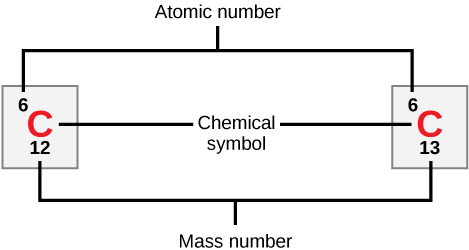2.1B: Atomic Number and Mass Number

•• Contributed by Boundless
• General Microbiology at Boundless

The atomic number is the number of protons in an element, while the mass number is the number of protons plus the number of neutrons.

Learning Objectives

• Determine the relationship between the mass number of an atom, its atomic number, its atomic mass, and its number of subatomic particles

Key Points

• Neutral atoms of each element contain an equal number of protons and electrons.
• The number of protons determines an element’s atomic number and is used to distinguish one element from another.
• The number of neutrons is variable, resulting in isotopes, which are different forms of the same atom that vary only in the number of neutrons they possess.
• Together, the number of protons and the number of neutrons determine an element’s mass number.
• Since an element’s isotopes have slightly different mass numbers, the atomic mass is calculated by obtaining the mean of the mass numbers for its isotopes.

Key Terms

• mass number: The sum of the number of protons and the number of neutrons in an atom.
• atomic number: The number of protons in an atom.
• atomic mass: The average mass of an atom, taking into account all its naturally occurring isotopes.

Atomic Number

Neutral atoms of an element contain an equal number of protons and electrons. The number of protons determines an element’s atomic number (Z) and distinguishes one element from another. For example, carbon’s atomic number (Z) is 6 because it has 6 protons. The number of neutrons can vary to produce isotopes, which are atoms of the same element that have different numbers of neutrons. The number of electrons can also be different in atoms of the same element, thus producing ions (charged atoms). For instance, iron, Fe, can exist in its neutral state, or in the +2 and +3 ionic states.

Mass Number

An element’s mass number (A) is the sum of the number of protons and the number of neutrons. The small contribution of mass from electrons is disregarded in calculating the mass number. This approximation of mass can be used to easily calculate how many neutrons an element has by simply subtracting the number of protons from the mass number. Protons and neutrons both weigh about one atomic mass unit or amu. Isotopes of the same element will have the same atomic number but different mass numbers.Atomic number, chemical symbol, and mass number: Carbon has an atomic number of six, and two stable isotopes with mass numbers of twelve and thirteen, respectively. Its average atomic mass is 12.11.

Scientists determine the atomic mass by calculating the mean of the mass numbers for its naturally-occurring isotopes. Often, the resulting number contains a decimal. For example, the atomic mass of chlorine (Cl) is 35.45 amu because chlorine is composed of several isotopes, some (the majority) with an atomic mass of 35 amu (17 protons and 18 neutrons) and some with an atomic mass of 37 amu (17 protons and 20 neutrons).

Given an atomic number (Z) and mass number (A), you can find the number of protons, neutrons, and electrons in a neutral atom. For example, a lithium atom (Z=3, A=7 amu) contains three protons (found from Z), three electrons (as the number of protons is equal to the number of electrons in an atom), and four neutrons (7 – 3 = 4).